# 目标检测

0.手工时代
1.RCNN
2.SPP
3.FAST-RCNN
4.FASTER-RCNN

## 评价标准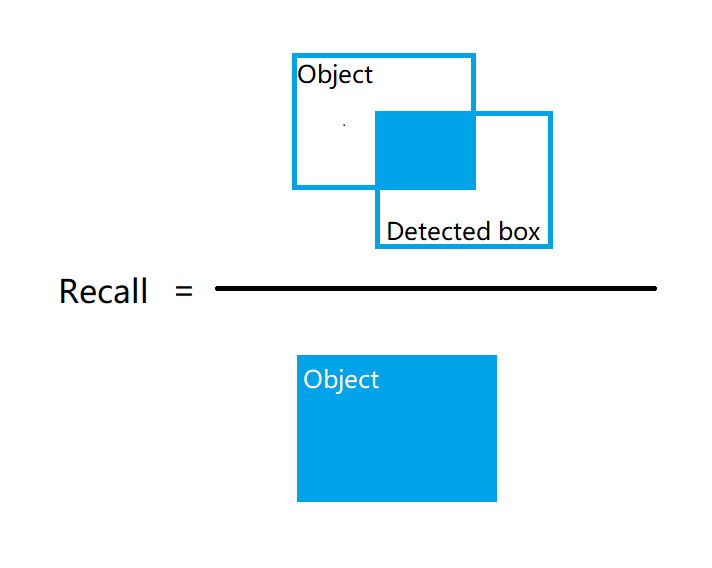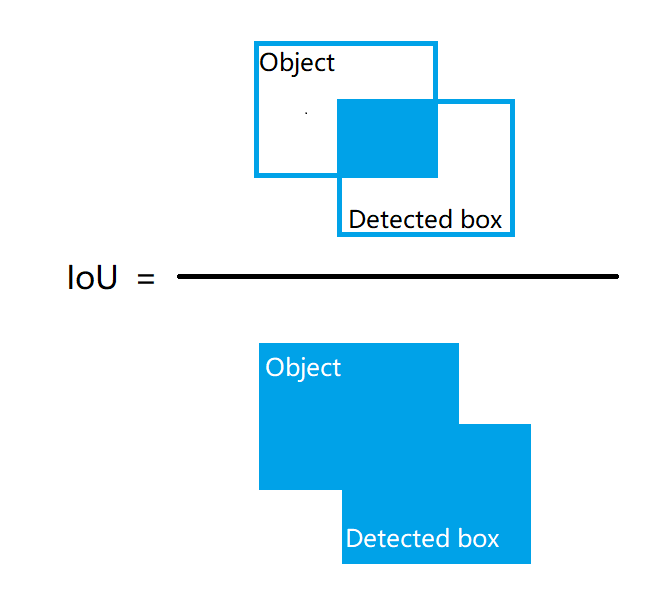P r e c i s o n c Precison_c :c在一张图片上识别准确率；
N ( T r u e P o s i t i v e s ) c N(TruePositives)_c :在一张图片上被识别出来C的个数；
N ( T o t a l O b j e c t s ) c N(TotalObjects)_c :在一张图片上C的个数；
A v e r a g e P r e c i s i o n c AveragePrecision_c :在整个数据集上C被识别的平均准确率；
∑ P r e c i s i o n c \sum{Precision_c} :在整个数据集上C被识别概率的总和；
N ( T o t a l I m a g e s ) c N(TotalImages)_c :数据集上有C的图片张数；
M e a n A v e r a g e P r e c i s i o n MeanAveragePrecision :数据集上所有类别的平均准确率；
∑ A v e r a g e P r e c i s i o n \sum{AveragePrecision} :数据集上所有类别平均准确率的总和；
N ( c l a s s e s ) N(classes) :数据集上有有类别的个数；
P r e c i s i o n C = N ( t r u e P o s i t i v e s ) c N ( T o t a l O b j e c t s ) c A v e r a g e P r e c i s i o n c = ∑ P r e c i s i o n c N ( T o t a l I m a g e s ) c M e a n A v e a r a g e P r e c i s i o n = ∑ A v e r a g e P r e c i s i o n c N ( c l a s s e s ) \begin{aligned} Precision_C &amp;= \frac{N(truePositives)_c}{N(TotalObjects)_c}\\ AveragePrecision_c &amp;= \frac{\sum{Precision_c}}{N(TotalImages)_c}\\ MeanAvearagePrecision &amp;= \frac{\sum{AveragePrecision_c}}{N(classes)} \end{aligned}

## RCNN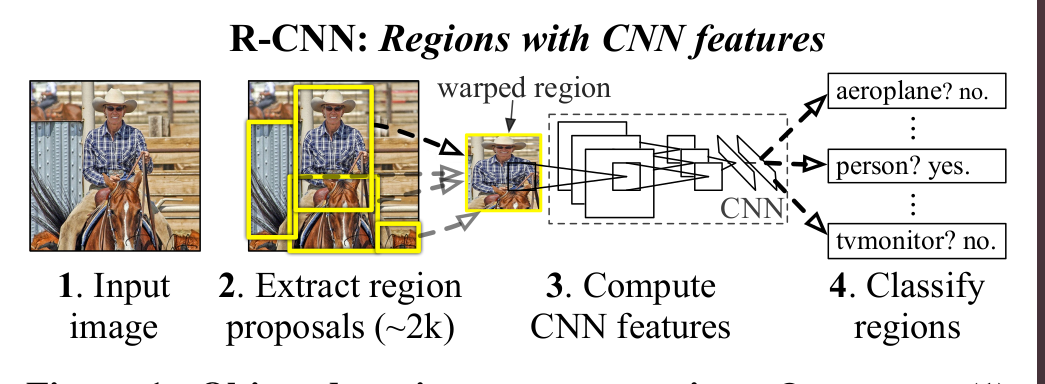1.下载模型进行微调<正样本IOU>0.5,负样本IOU<0.5>
2.使用SS算法选取候选区域
3.将候选区域内的图形变形作为网络输入，同时将网络输出的第5层特征保存下来
4.将上步保存的特征作为SVM的输入进行训练<SVM训练的正负样本，正样本：ground-truth内的样本；负样本：IOU<0.3 &其他样本的IOU<0.7>
5.将第三步保存的特征作为输入，进行bounding-box进行回归学习<输入 ( P x , P y , P w , P h ) (P_x,P_y,P_w,P_h) CNN 特征，输出： ( d x ( P ) , d y ( P ) , d w ( P ) , d h ( P ) ) (d_x(P),d_y(P),d_w(P),d_h(P)) >；

## SPP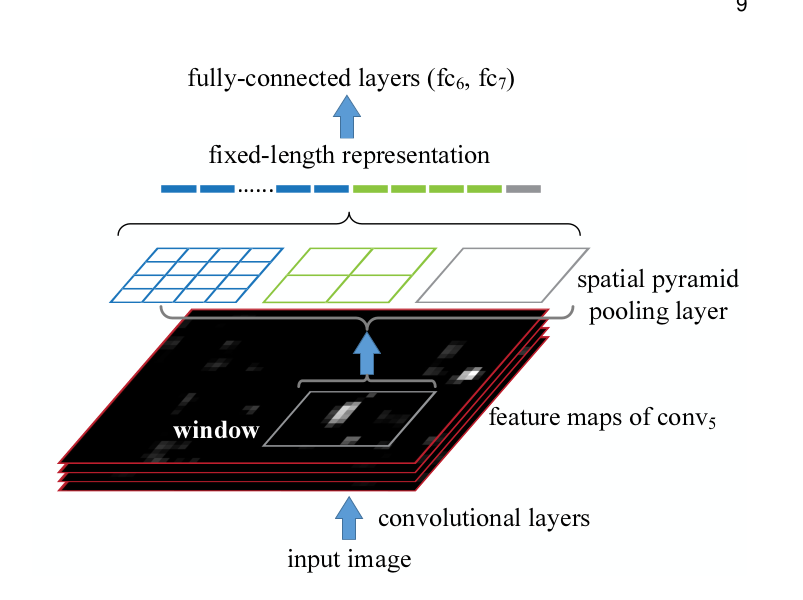1.下载模型进行微调
2.使用CONV层提取特征&使用SS算法选取候选区域
3.将SS算法选取的候选区域映射到conv特征提取层
4.对相应区域的特征提取层输入到spatial pyramid pooling layer层提取固定长度的特征
5.将固定长度的特征输入到全连接层，最后进行SVM分类
6.将全连接层的输出，进行bounding-box的学习

## FAST-RCNN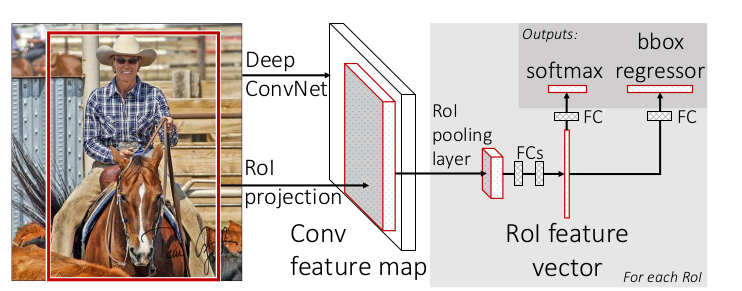1.下载模型进行微调
2.使用CONV层提取特征&使用SS算法选取候选区域
3.将SS算法选取的候选区域映射到conv特征提取层
4.对相应区域的特征提取层输入到ROI层提取固定长度的特征
5.将固定长度的特征输入到全连接层，最后进行Softmax分类
6.将全连接层的输出，进行bounding-box的学习

## FASTER-RCNN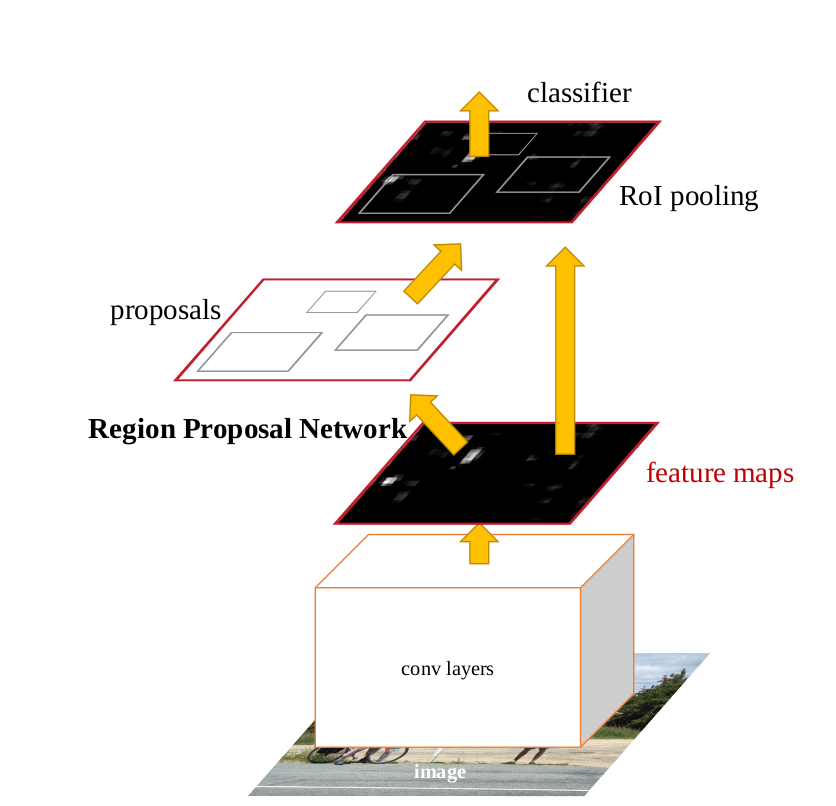1.下载模型进行微调
2.从conv层提取的特征训练RPN<用于候选区域的生成>网络；
3.使用ROI层提取固定长度的特征
4.在2学习到的网络上继续进行分类和bounding-box的回归；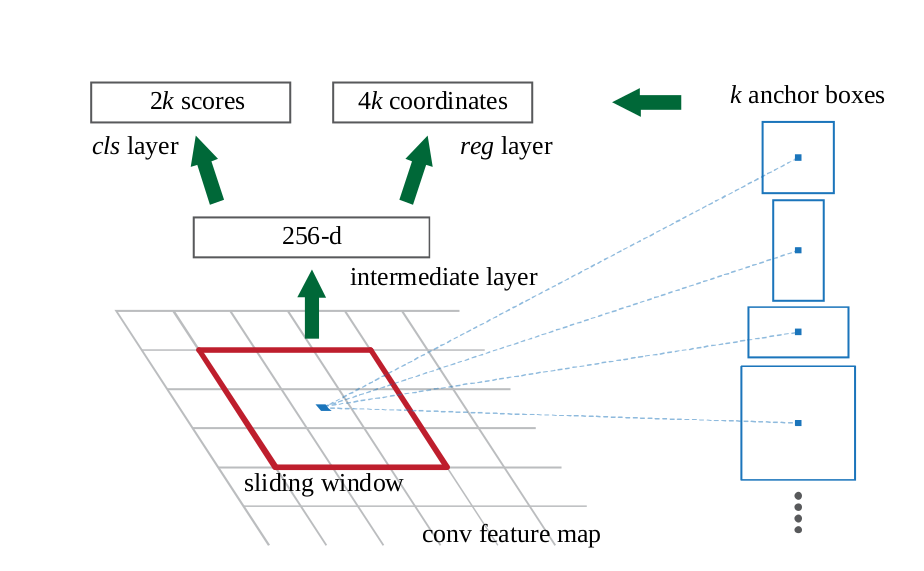12-02
04-2708-204万+
07-096万+
06-23
06-102万+
11-221万+
10-121万+
08-251万+